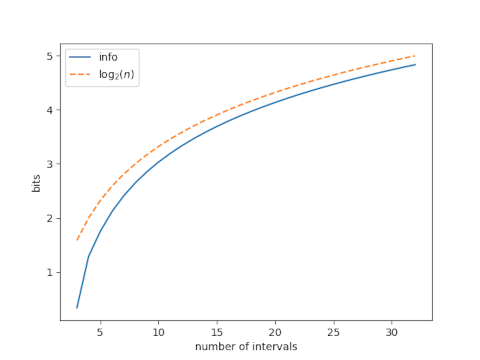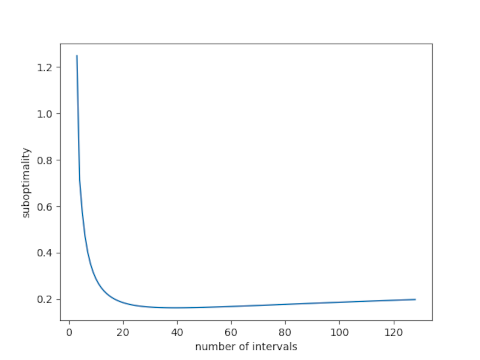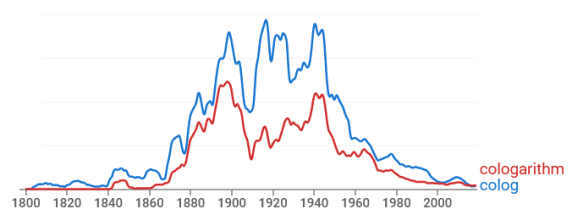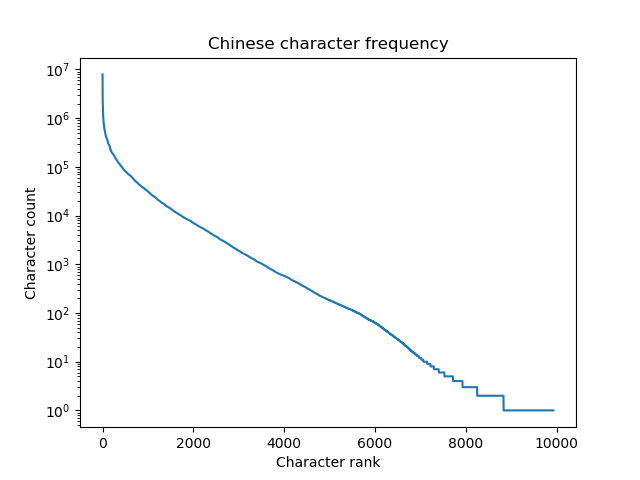# Logarithms yearning to be free

I got an evaluation copy of The Best Writing on Mathematics 2021 yesterday. One article jumped out as I was skimming the table of contents: A Zeroth Power Is Often a Logarithm Yearning to Be Free by Sanjoy Mahajan. Great title.

There are quite a few theorems involving powers that have an exceptional case that involves a logarithm. The author opens with the example of finding the antiderivative of xn. When n ≠ -1 the antiderivative is another power function, but when n = -1 it’s a logarithm.

Another example that the author mentions is that the limit of power means as the power goes to 0 is the geometric mean. i.e. the exponential of the mean of the logarithms of the arguments.

I tried to think of other examples where this pattern pops up, and I thought of a couple related to entropy.

## q-logarithm entropy

The definition of q-logarithm entropy takes Mahajan’s idea and runs it backward, turning a logarithm into a power. As I wrote about here,

The natural logarithm is given byand we can generalize this to the q-logarithm by definingAnd so ln1 = ln.

Then q-logarithm entropy is just Shannon entropy with natural logarithm replaced by q-logarithm.

## Rényi entropy

Quoting from this post,

If a discrete random variable X has n possible values, where the ith outcome has probability pi, then the Rényi entropy of order α is defined to befor 0 ≤ α ≤ ∞. In the case α = 1 or ∞ this expression means the limit as α approaches 1 or ∞ respectively.

When α = 1 we get the more familiar Shannon entropy:In this case there’s already a logarithm in the definition, but it moves inside the parentheses in the limit.

And if you rewrite

pα

as

p pα-1

then as the exponent in pα-1 goes to zero, we have a logarithm yearning to be free.

# Information in a discretized normal

Here’s a problem that came up in my work today.

Suppose you have a normal random variable X and you observe X in n discrete bins. The first bin is the left α tail, the last bin is the right α tail, and the range between the two tails is divided evenly into n-2 intervals. How many bits of information are in such an observation?

For example, assume adult male heights are normally distributed with mean 70 inches and standard deviation 3 inches. How much information is there in an observation of height, rounded down to the nearest inch, if we put all heights less than 64 inches in a bin and all heights greater than or equal to 76 inches in a bin?

Here α = 0.025 because that’s the probability that a normal random variable is more than 2 standard deviations below its mean or more than 2 standard deviations above its mean.

We have n = 15 because we have intervals (-∞, 64), [64, 65), [65, 66), … [75, 76), and [76, ∞).

We know that with n bins we have at most log2n bits of information. That would correspond to n bins of equal probability. In other words, we’d get the maximum information if we spaced our bins evenly in terms of percentiles rather in terms of inches.

So how does the information content vary as a function of the number of bins, and how close is it to the maximum information for n bins?

Here’s a plot, with α = 0.025.The suboptimality of our bins, i.e. the difference between log2n and the information we get from n bins, drops quickly at first as n increases, then appears to plateau.

Next lets look at just the suboptimality, and increase our range of n.This shows that the suboptimality does not approach an asymptote but instead starts to increase. The minimum at n = 36 is 0.16 bits.

# Information loss and entropy

John Baez, Tobias Fritz, and Tom Leinster wrote a nice paper  that shows Shannon entropy is the only measure of information loss that acts as you’d expect, assuming of course you have the right expectations. See their paper for all the heavy lifting. All I offer here is an informal rewording of the hypotheses.

The authors say that

We show that Shannon entropy gives the only concept of information loss that is functorial, convex-linear and continuous.

You could reword this by saying

Shannon entropy is the only measure of information loss that composes well, mixes well, and is robust.

Saying that a measure of information loss composes well means that the information lost by doing two processes f and then g is the sum of the information lost by doing f and the information lost by doing g. This is what the authors describe as functorial.

When I refer to something mixing well, I have in mind mixtures in the sense of probability. A mixture is a random choice of random variables, such as flipping a coin to decide which of two dice to roll.

Going back to our two processes f and g, if we choose to do f with probability p and to do g with probability (1 – p) then the information loss from this mixture process should be p times the information loss from f plus (1-p) times the information lost from g. Instead of saying this mixes well, you could say it behaves as expected, where “as expected” is a pun on expected value. The authors describe this as convex-linear because the expected information loss is a convex combination of the two possible losses.

Robustness is a more colloquial term for continuity. Something is robust if small changes in inputs should lead to small changes in outputs. If you make this statement rigorous, you end up with the definition of continuity. If we change a process f a little then we should change our measure of information loss a little.

## More on information theory

 A characterization of entropy in terms of information loss. On arXiv.

# Index of coincidence

Index of coincidence is a statistic developed by William Friedman for use in cryptanalysis. It measures how unevenly symbols are distributed in a message.

It’s a kind of signature that could be used, for example, to infer the language of a text, even if the text has been encrypted with a simple substitution cipher. It also has more sophisticated uses, such as inferring key length in text that has been encrypted with a Vigenère cipher.

If a discrete random variable has n possible values, each occurring with probability pi, then its index of coincidence isIn cryptanalysis, the probabilities are the letter frequencies in the language of the message. The sum above is often multiplied by some constant, but this makes no difference in application because you would compare index of coincidence values, not interpret the index in the abstract.

Often the sum above is multiplied by n. This amounts to dividing the sum above by the corresponding sum for a uniform distribution.

Index of coincidence is occasionally use in applications, though not in cryptography anymore.

## Example

The letter frequencies in Google’s English language corpus are given here. In this corpus the probabilities range from 0.1249 for e down to 0.0009 for z. The index of coincidence would be

0.1249² + 0.0928² + … + 0.0009² = 0.06612

You’re more likely to see this value multiplied by 26, which gives 1.719.

## Relation to entropy

Index of coincidence seems a lot like entropy, and in fact it is a simple transformation of an entropy, though not Shannon entropy. Index of coincidence is closely related to Rényi entropy.

Rényi entropy of order α is defined for a random variable X to beand so Rényi entropy of order 2 is the negative log of the index of coincidence. That is, if H is the Rényi entropy of order 2 and I is the index of coincidence, thenIndex of coicidence and Rényi entropy are sometimes defined with multiplicative constants that would slightly change the equations above.

Since exp(-x) is a decreasing function, increasing index of coincidence corresponds to decreasing Rényi entropy. Sorting in increasing order by index of coincidence is the same as sorting in decreasing order by Rényi entropy.

# Information theory and coordinates

The previous post explains how the Maidenhead location system works. The first character in a location code specifies the longitude in 20 degree increments and the second character specifies the latitude in 10 degree increments. Both are a letter from A through R that breaks the possible range down into 18 parts. (The longitude range is wider because longitude ranges over 360 degrees but latitude ranges over 180 degrees.)

If you divide a range into 16 equally probable parts, you get four bits of information because 24 = 16. Since we’re dividing longitude and latitude into 18 parts, we get around 4 bits of information from each. But the longitude component carries more information than the latitude component. This post will explain why.

## Defining information

The Shannon entropy of a random variable with N possible values, each with probability pi, is defined to bewhere the base b is usually 2. Occasionally b might be another base, such as 2 or 10. (More on that here.) The statement above about 16 equal parts giving 4 bits of information assumes b = 2.

The expected information gain from observing the value of a random variable is measured by the random variable’s Shannon entropy.

## Even vs uneven allocation

Suppose we pick a point at random on a sphere. The first component of the point’s Maidenhead location narrows the location of the point to one of 18 equally probable sectors. So each of the ps in the equation above is 1/18. That means the information content in the first component is log2(1/18) = 4.17 bits.

The second component of the location also divides the sphere into 18 parts, but not evenly. A 10 degree band of latitude near the equator covers more area than a 10 degree band of latitude near the polls.

Shannon entropy is maximized when all the ps are equal. Said another way, the amount of surprise from observing a random variable is greatest when all possibilities are equally likely.

Knowing that a random point on a sphere is within 10 degrees of the equator is not as surprising as knowing that it is within 10 degrees of the North Pole because there’s less land within 10 degrees of the North Pole.

## Archimedes and Shannon

To calculate the information content in the latitude band, we need to know the area of each band.

Archimedes (c. 287 – 212 BC) discovered that if you have a sphere sitting inside a cylinder, the area of a band on the sphere is proportional to the area of its projection onto the cylinder. That means the area of a band of latitude is proportional to the difference in the z coordinates of the band.

The 18 bands of latitude run from -90° to 90° in increments of 10°. If we number the bands starting with 1 at the South Pole, the probability of landing in the nth band is

(sin(-90° + 10n°) – sin(-90° + 10(n-1)°)/2

and you can calculate from this that the Shannon entropy is 3.97.

So the first component of the location, the longitude, carries 4.17 bits of information, and the second component, the latitude, carries 3.97 bits.

# Cologarithms and Entropy

The term “cologarithm” was once commonly used but now has faded from memory. Here’s a plot of the frequency of the terms cololgarithm and colog from Google’s Ngram Viewer.The cologarithm base b is the logarithm base 1/b, or equivalently, the negative of the logarithm base b.

cologb x = log1/b x = -logb x

I suppose people spoke of cologarithms more often when they did calculations with logarithm tables.

## Entropy

There’s one place where I would be tempted to use the colog notation, and that’s when speaking of Shannon entropy.

The Shannon entropy of a random variable with N possible values, each with probability pi, is defined to beAt first glace this looks wrong, as if entropy is negative. But you’re taking the logs of numbers less than 1, so the logs are negative, and the negative sign outside the sum makes everything positive.

If we write the same definition in terms of cologarithms, we havewhich looks better, except it contains the unfamiliar colog.

## Bits, nats, and dits

These days entropy is almost always measured in units of bits, i.e. using b = 2 in the definition above. This wasn’t always the case.

When logs are taken base e, the result is in units of nats. And when the logs are taken base 10, the result is in units of dits.

So binary logs give bits, natural logs give nats, and decimal logs give dits.

Bits are sometimes called “shannons” and dits were sometimes called “bans” or “hartleys.” The codebreakers at Bletchley Park during WWII used bans.

The unit “hartley” is named after Ralph Hartley. Hartley published a paper in 1928 defining what we now call Shannon entropy using logarithms base 10. Shannon cites Hartley in the opening paragraph of his famous paper A Mathematical Theory of Communication.

# Chinese character frequency and entropy

How much information is conveyed by a single Chinese character? This post addresses that question by computing Shannon entropy.

First of all, information theory defines the Shannon entropy of an “alphabet” to bebits where pi is the probability of the ith “letter” occurring and the sum is over all letters. I put alphabet and letter in quotes because information theory uses these terms for any collection of symbols. The collection could be a literal alphabet, like the Roman alphabet, or it could be something very different, such as a set of musical notes. For this post it will be a set of Chinese characters.

I’ll use a set of data on Chinese character frequency by Jun Da. See that site for details.

Here’s a plot of the character frequencies on a logarithmic scale.Jun Da’s data set contains 9,933 characters. The most common character, 的, appears 7,922,684 times in the language corpus and the least common character on the list, 鴒, appears once. The 1,000 most common characters account for 89% of use.

The Shannon entropy of the Chinese “alphabet” is 9.56 bits per character. By comparison, the entropy of the English alphabet is 3.9 bits per letter.

The entropy of individual symbols doesn’t tell the whole story of the information density of the two writing systems. Symbols are not independent, and so the information content of a sequence of symbols will be less than just multiplying the information content per symbol by the number of symbols. I imagine there is more sequential correlation between consecutive English letters than between consecutive Chinese characters, though I haven’t seen any data on that. If this is the case, just looking at the entropy of single characters underestimates the relative information density of Chinese writing.

Although writing vary substantially in how much information they convey per symbol, spoken languages may all convey about the same amount of information per unit of time.

A few weeks ago I wrote about new research suggesting that all human languages convey information at about the same rate. Some languages carry more information per syllable than others, as measured by Shannon entropy, but these languages are typically spoken more slowly. The net result is that people convey about 40 bits of information per second, independent of their language. Of course there are variations by region, by individual, etc. And there are limits to any study, though the study in question did consider a wide variety of languages.

# Entropy extractor used in μRNG

Yesterday I mentioned μRNG, a true random number generator (TRNG) that takes physical sources of randomness as input. These sources are independent but non-uniform. This post will present the entropy extractor μRNG uses to take non-uniform bits as input and produce uniform bits as output.

We will present Python code for playing with the entropy extractor. (μRNG is extremely efficient, but the Python code here is not; it’s just for illustration.) The code will show how to use the pyfinite library to do arithmetic over a finite field.

## Entropy extractor

The μRNG generator starts with three bit streams—X, Y, and Z—each with at least 1/3 bit min-entropy per bit.

Min-entropy is Rényi entropy with α = ∞. For a Bernoulli random variable, that takes on two values, one with probability p and the other with probability 1-p, the min-entropy is

-log2 max(p, 1-p).

So requiring min-entropy of at least 1/3 means the two probabilities are less than 2-1/3 = 0.7937.

Take eight bits (one byte) at a time from XY, and Z, and interpret each byte as an element of the finite field with 28 elements. Then compute

X*YZ

in this field. The resulting stream of bits will be independent and uniformly distributed, or very nearly so.

## Purified noise

Just a quick aside. Normally you want to remove noise from data to reveal a signal. Said another way, you want to split the data into signal and noise so you can throw out the noise. Here the goal is the opposite: we want to remove any unwanted signal in order to create pure noise!

## Python implementation

We will need the bernoulli class for generating our input bit streams, and the pyfinite for doing finite field arithmetic on the bits.

    from scipy.stats import bernoulli
from pyfinite import ffield


And we will need a couple bit manipulation functions.

    def bits_to_num(a):
"Convert an array of bits to an integer."

x = 0
for i in range(len(a)):
x += a[i]*2**i
return x

def bitCount(n):
"Count how many bits are set to 1."
count = 0
while(n):
n &= n - 1
count += 1
return count


The following function generates random bytes using the entropy extractor. The input bit streams have p = 0.79, corresponding to min-entropy 0.34.

    def generate_byte():
"Generate bytes using the entropy extractor."

b = bernoulli(0.79)

x = bits_to_num(b.rvs(8))
y = bits_to_num(b.rvs(8))
z = bits_to_num(b.rvs(8))

F = ffield.FField(8)


Note that 79% of the bits produced by the Bernoulli generator will be 1’s. But we can see that the output bytes are about half 1’s and half 0’s.

    s = 0
N = 1000
for _ in range(N):
s += bitCount( generate_byte() )
print( s/(8*N) )


This returned 0.50375 the first time I ran it and 0.49925 the second time.

For more details see the μRNG paper.

## Update: RNG test suite results

I ran an experiment, creating streams of biased data and running them through the entropy extractor. The first post in the series, NIST STS, explains the set up. The last post in the series, using TestU01, summarizes the results. In a nutshell, the extractor passes STS and DIEHARDER, but fails PractRand and TestU01.

# Solving for probability given entropy

If a coin comes up heads with probability p and tails with probability 1-p, the entropy in the coin flip is

S = –p log2 p – (1-p) log2 (1-p).

It’s common to start with p and compute entropy, but recently I had to go the other way around: given entropy, solve for p. It’s easy to come up with an approximate solution.Entropy in this case is approximately quadratic

S ≈ 4p(1-p)

and so

p ≈ (1 ± √(1-S))/2.

This is a good approximation if S is near 0 or 1 but mediocre in the middle. You could use solve for p numerically, say with Newton’s method, to get more accuracy if needed.

## Update

As Sjoerd Visscher pointed out in the comments, the quadratic approximation for entropy is much better if you raise it to the power 3/4. When I added this new approximation to the graph above, the new approximation agreed with the correct value to within the thickness of the plotting line.

To make the approximation error visible, here’s the log of the absolute value of the error of the two approximations, on a log scale.The error in the new approximation is about an order of magnitude smaller, sometimes more.

The improved approximation for entropy is

S ≈ (4p(1-p))3/4

and so the new approximation for probability is

p ≈ (1 ± √(1-S4/3))/2.

# Levenshtein distance from Finnegans Wake to Return of the JediI ran into a delightfully strange blog post today called Finnegans Ewok that edits the first few paragraphs of Finnegans Wake to make it into something like Return of the Jedi.

(Unfortunately the page has gone away since I first wrote this. Some of the text is preserved in this Python script.)

The author, Adam Roberts, said via Twitter “What I found interesting here was how little I had to change Joyce’s original text. Tweak a couple of names and basically leave it otherwise as was.”

So what I wanted to do is quantify just how much had to change using the Levenshtein distance, which is essentially the number of one-character changes necessary to transform one string into another.

Here’s the first paragraph from James Joyce:

riverrun, past Eve and Adam’s, from swerve of shore to bend of bay, brings us by a commodius vicus of recirculation back to Howth Castle and Environs.

And here’s the first paragraph from Adam Roberts:

movierun, past new and hopes, from strike of back to bend of jeday, brings us by a commodius lucas of recirculation back to forestmoon and endor.

The original paragraph is 150 characters, the parody is 145 characters, and the Levenshtein distance is 44.

Here’s a summary of the results for the first four paragraphs.

    |-------+---------+----------|
| Joyce | Roberts | Distance |
|-------+---------+----------|
|   150 |     145 |       44 |
|   700 |     727 |      119 |
|   594 |     615 |      145 |
|  1053 |     986 |      333 |
|-------+---------+----------|


The fifth paragraph seems to diverge more from Joyce. I maybe have gotten something misaligned, and reading enough of Finnegans Wake to debug the problem made my head hurt, so I stopped.

Update: See the next post for sequence alignment applied to the two sources. This lets you see not just the number of edits but what the edits are. This show why I was having difficulty aligning the fifth paragraphs.